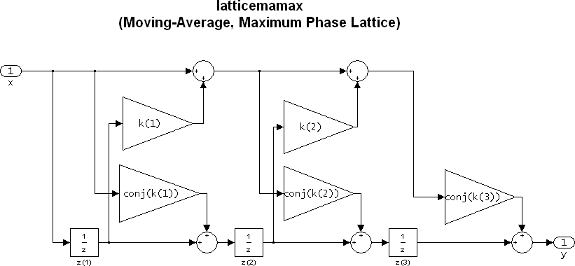Documentation

### This is machine translation

Mouseover text to see original. Click the button below to return to the English version of the page.

To view all translated materials including this page, select Country from the country navigator on the bottom of this page.

# dfilt.latticemamax

Discrete-time, lattice, moving-average filter

## Syntax

```Hd = dfilt.latticemamax(k) Hd = dfilt.latticemamax ```

## Description

`Hd = dfilt.latticemamax(k)` returns a discrete-time, lattice, moving-average filter, `Hd`, with lattice coefficients `k`.

### Note

If the `k` coefficients define a maximum phase filter, the resulting filter in this structure is maximum phase. If your coefficients do not define a maximum phase filter, placing them in this structure does not produce a maximum phase filter.

`Hd = dfilt.latticemamax` returns a default discrete-time, lattice, moving-average filter, `Hd`, with `k`=[ ]. This filter passes the input through to the output unchanged.The resulting filter states column vector is

`$\left[\begin{array}{c}z\left(1\right)\\ z\left(2\right)\\ z\left(3\right)\end{array}\right]$`

## Examples

Form a fourth-order lattice, moving-average, maximum phase filter structure for a `dfilt` object, `Hd`, using the following lattice coefficients:

```k = [.66 .7 .44 .33]; Hd = dfilt.latticemamax(k) ```